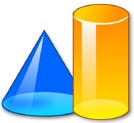Explore the amazing world of math for kids and get help online with our collection of fun math problems, cool worksheets, interactive activities and other free resources. Find games, videos, quizzes, facts and puzzles that will help motivate kids to improve their math skills. Learn about addition, subtraction, multiplication, division, fractions, decimals, numbers, geometry, shapes, angles, probability and other interesting math topics. Enjoy activities for kids, ideas for parents and handy resources for teachers that make learning about mathematics fun! Sponsored Links

 Sponsored LinksLearn about a range of math topics with these fun math games for kids. Solve equations, improve your arithmetic, complete geometry based challenges, experiment with numbers, learn about statistics and much more.Addition Brush up on your addition skills with this interactive math game for kids that challenges you to build a giant water slide by adding numbers together.Multiplication Solve a range of interactive multiplication problems with this educational math game for kids.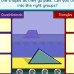Shapes How well do you know your shapes? Find out with this cool activity that tests your knowledge of geometric and 3D shapes.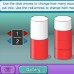Fractions Learn about fractions by completing a range of fun challenges with this great math game for kids.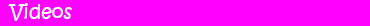Take a look at these cool math videos. Enjoy the numbers of nature, learn about basic geometric shapes and more.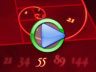Numbers of Nature Enjoy this inspiring video that features numbers found in nature.Learn Basic Shapes Learn about circles, triangles, squares, cylinders and other basic shapes;Funny Multiplication Trick This funny math video is sure to bring a smile to your face.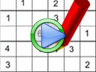How to Solve Sudoku Puzzles Learn how to solve sudoku puzzles with this handy video.Put your math knowledge to the test by trying one of our fun quizzes. Answer a range of interesting questions related to addition, subtraction, multiplication, geometry, statistics, prime numbers and more.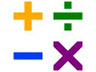Give our fun math quiz for kids a try and see how many of the interesting math questions you can answer correctly.Geometry Quiz for Kids Answer questions related to angles, dimensions, measurements and shapes with our geometry quiz for kids.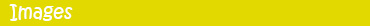Check out our range of free math pictures, photos, images, diagrams and clip art. Find interesting shapes, symbols, numbers and more.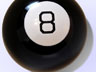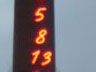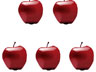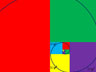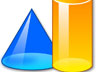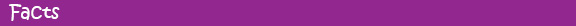Check out our range of fun math facts for kids and learn about amazing numbers, unique shapes, the golden ratio, math history and more.Math Facts Enjoy some cool math facts and learn interesting trivia related to a range of math topics. How many decimal places do you know the value of pi to? Do you know what a 'googol' is? How about a 'googolplex'? How many pieces can you cut a cake into with just 3 slices?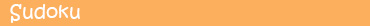Put your problem solving skills to the test with our range of printable sudoku puzzles for kids. Start with the basics or get straight into brain bendingly difficult puzzles.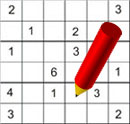Sudoku Puzzles Improve your number skills with these fun sudoku puzzle worksheets that range in difficulty from easy through to medium and difficult. How many can you complete?Science Kids ©  |  Home  |  About  |  Topics  |  Experiments  |  Games  |  Facts  |  Quizzes  |  Projects  |  Lessons  |  Images  |  Videos  |  Privacy  |  Sitemap  |  Updated: Apr 11, 2020